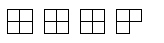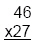Name:    3rd Grade Math Proficient Test 2

Multiple Choice
Identify the choice that best completes the statement or answers the question.

1.

Computation – Fractions – RIT 191 – 200

Which of these fractions is equal to 1?
 a. 7/4 d. 6/6 b. 4/2 e. 2/3 c. 5/6

2.Which fraction correctly names the above picture?
 a. 15/4 d. 16/4 b. 20/4 e. 4/4 c. 1/4

3.

5/7 – 3/7 =
 a. 8/7 d. 0 b. 2 e. 7 c. 2/7

4.

1/3 + 1/2 =
 a. 2/5 d. 5/6 b. 10/12 e. 2/6 c. 1/5

5.

Computation – Percents – RIT 191 – 200

What is 4% of 20?
 a. 24 d. 0.04 b. 16 e. 0.8 c. 8

6.

Computation – Whole Numbers – RIT 191 – 200
A person bought 33 apples and 14 pears at a store. How many items did the
 a. 33 d. 19 b. 47 e. 46 c. 57

7.

Find the pattern and answer the question.
5, 11, 17, 23, 29, 35, 41, 47
Which number would correctly fit the pattern?
 a. 47 d. 53 b. 5 e. 48 c. 49

8.

(7 + 2) + 3 = 7 + (2 +___  )
 a. 3 d. 0 b. 2 e. 12 c. 7

9.

Which equation illustrates the commutative property of addition?
 a. 3 + 2 + 1 = 6 d. 4 – 3 = 1 b. 3 + 2 = 2 + 3 e. 2 + 3 = 1 + 4 c. 2 x 3 = 2 + 4

10.

Lisa threw five balls at a target. She picked up the balls and threw all of them
at the target again. How many times did Lisa throw?
 a. 5 d. 7 b. 10 e. 15 c. 2

11.

27,049
-13,927
 a. 40,976 d. 13,122 b. 13,132 e. 14,122 c. 14,922

12.

Round to the nearest hundred.
43,362
 a. 43,400 d. 43,300 b. 43,362 e. 43,360 c. 40,000

13.

23 X 13 =
 a. 296 d. 179 b. 36 e. 299 c. 409

14.46
x 27
 a. 73 d. 1,102 b. 1,242 e. 1,112 c. 19

15.

7,964
-5,821
 a. 2,243 d. 2,043 b. 13,785 e. 3,143 c. 2,143

16.

729 X 56 =
 a. 39,274 d. 8,019 b. 3,645 e. 40,824 c. 785

17.

7,040 X 103 =
 a. 7,143 d. 91,520 b. 28,622 e. 727,114 c. 725,120

18.

Computation – Decimals – RIT 201 –210

5.01 + 23.4 =
 a. 28.5 d. 7.35 b. 29 e. 28.41 c. 53.05

19.

9.62
- 3.51
 a. 13.13 d. 6.11 b. 6.1 e. 12.1 c. 5.1

20.

\$50.00 - \$10.25 =
 a. \$60.25 d. \$40.75 b. \$39.75 e. \$49.25 c. \$40.25

21.

0.17658 - 0.04237 =
 a. 0.07428 d. .21895 b. 1.17488 e. .051929 c. 0.13421